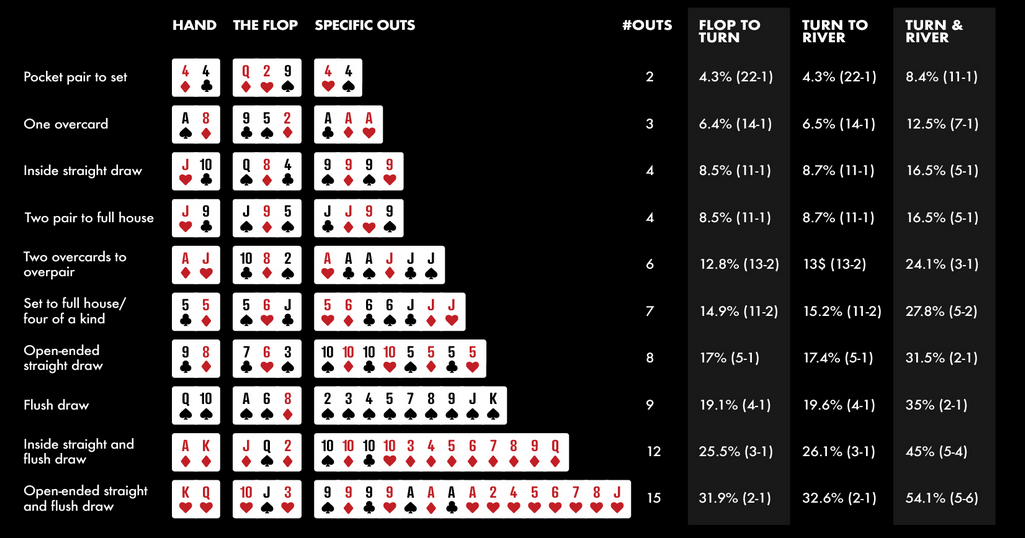Poker is not just a game of luck; it also involves skill and mathematics. Understanding poker math and being able to calculate odds and pot odds accurately can greatly improve your chances of winning. In this article, we will explore the concept of poker math and break down how to calculate odds and pot odds effectively.

## Understanding Poker Odds

Poker odds refer to the probability of winning a hand based on the cards you have and the cards on the table. It allows you to mathematically analyze the strength of your hand and make informed decisions based on the likelihood of winning. To calculate poker odds, you need to consider the number of possible outcomes and the number of favorable outcomes.

### Calculating Pre-Flop Odds

Pre-flop odds refer to the probability of getting a specific hand before the flop, without taking into account the community cards. To calculate pre-flop odds, you need to know the total number of possible hand combinations and the number of specific hands you want to achieve. For example, if you want to calculate the odds of getting pocket aces (the best starting hand), you would divide the number of possible pocket card combinations (6, 45) by the total number of hand combinations (1, 2).

### Calculating Post-Flop Odds

Post-flop odds are calculated after the flop, when the community cards are revealed. Now, you have more information to work with and can calculate the odds of improving your hand further. To calculate post-flop odds, you need to consider the number of outs, which are the remaining cards in the deck that can improve your hand, and the number of unknown cards. By dividing the number of outs by the number of unknown cards, you can determine the probability of hitting your desired card on the turn or river.

## Understanding Pot Odds

Pot odds, on the other hand, refer to the ratio between the current size of the pot and the cost of a contemplated call. It helps you decide whether a call or a bet is profitable in the long run. If the pot odds are greater than the odds of completing your hand, it is generally a favorable decision to make. However, if the pot odds are lower, it may be better to fold.

### Calculating Pot Odds

To calculate pot odds, you need to know the size of the current pot and the amount you need to call to continue playing. By dividing the cost of the call by the total pot size (including opponents’ bets), you can determine the pot odds. For example, if the pot is \$100 and you need to call \$20, the pot odds are 5:1. If the odds of completing your hand are 4:1, it would be profitable to make the call, as the pot odds are higher.

## Conclusion

Mastering poker math is essential for any serious poker player. By understanding how to calculate odds and pot odds, you can make more informed decisions and improve your overall win rate. Remember, poker is not just a game of luck; it is a game of skill and mathematics. So, take the time to practice and familiarize yourself with the concept of poker math. Happy playing!